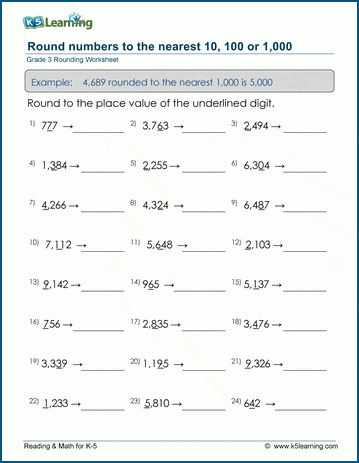# Grade 3 Place Value and Rounding Worksheets

As early as kindergarten students work on place value. By grade 3, students have progressed to building three, four and five-digit numbers from their parts, writing these numbers in expanded form, comparing and ordering numbers, skip counting by 100, 150, 200 and 250, as well as rounding numbers to the nearest 10, 100 and 1000.

## What is place value?

Let’s say we have a three-digit number, each number has a value 10 times that of the number on its right. Visually that looks like this: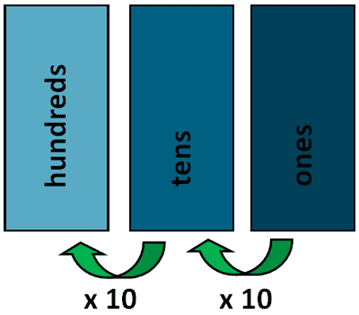For example, the number 472 would break down as follows: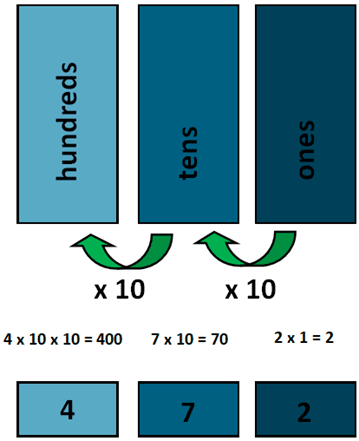## Place Value and Rounding Worksheets for Grade 3

We’ve created a whole section of worksheets for students to practice place value and rounding at the grade 3 level.

Let us cherry-pick among some of our favorite place value and rounding worksheets.

## Missing place values from 3-digit numbers

Students use a full three-digit number to locate the missing place value in its expanded form.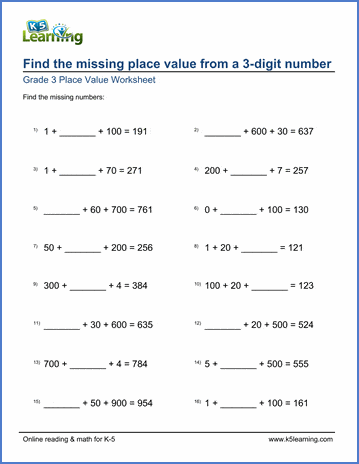## Build 5-digit numbers from their parts

Students rewrite 5-digit numbers from their expanded forms in these worksheets.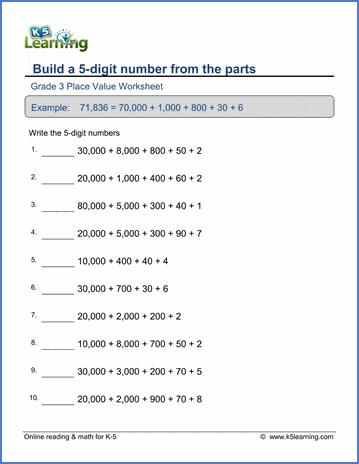## Write the expanded form of 4-digit numbers

In reverse of the above worksheets, students write 4-digit numbers in their expanded form.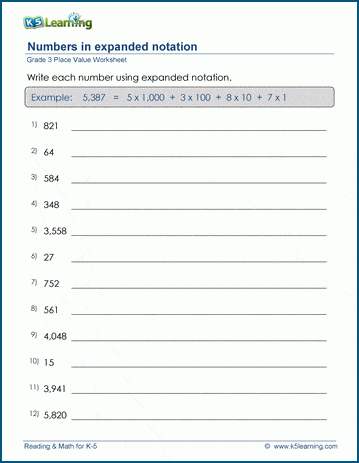## Ordering numbers up to 10,000 practice

Students are asked to order groups of numbers from the smallest to the largest number.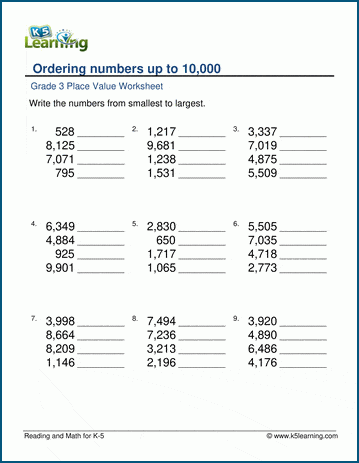## Skip counting by 150, 200 and 250 worksheets

Kids fill in the missing numbers in skip counting by 150, 200 and 250 on three separate worksheets.## Rounding 4-digit numbers to the nearest 1,000

Students practice rounding 4-digit numbers to the nearest 1,000.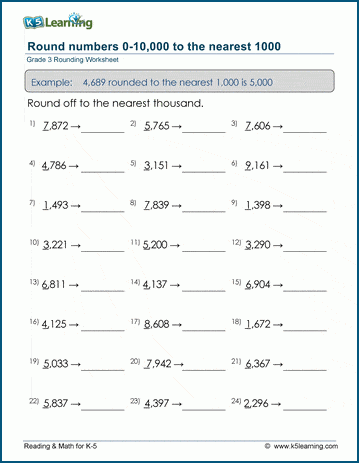## Mixed rounding worksheets

Kids round numbers to the nearest 10, 100 and 1,000 in these mixed rounding worksheets.